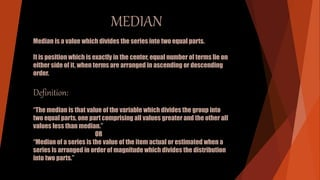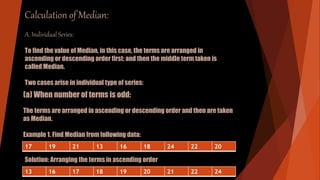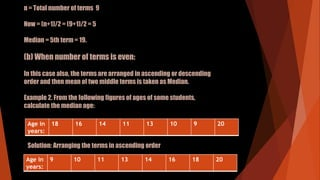Se está descargando tu SlideShare. ×Cargando en…3
×

1 de 22
1 de 22

# Median

how to calculate median.
merits and demerits of Median

how to calculate median.
merits and demerits of Median

### Median

1. 1. MEDIAN Median is a value which divides the series into two equal parts. It is position which is exactly in the center, equal number of terms lie on either side of it, when terms are arranged in ascending or descending order. Definition: “The median is that value of the variable which divides the group into two equal parts, one part comprising all values greater and the other all values less than median.” OR “Median of a series is the value of the item actual or estimated when a series is arranged in order of magnitude which divides the distribution into two parts.”
2. 2. Calculation of Median: A. Individual Series: To find the value of Median, in this case, the terms are arranged in ascending or descending order first; and then the middle term taken is called Median. Two cases arise in individual type of series: (a) When number of terms is odd: The terms are arranged in ascending or descending order and then are taken as Median. Example 1. Find Median from following data: 17 19 21 13 16 18 24 22 20 13 16 17 18 19 20 21 22 24 Solution: Arranging the terms in ascending order
3. 3. n = Total number of terms 9 Now = (n+1)/2 = (9+1)/2 = 5 Median = 5th term = 19. (b) When number of terms is even: In this case also, the terms are arranged in ascending or descending order and then mean of two middle terms is taken as Median. Example 2. From the following figures of ages of some students, calculate the median age: Age in years: 18 16 14 11 13 10 9 20 Solution: Arranging the terms in ascending order Age in years: 9 10 11 13 14 16 18 20
4. 4. Now, median age =[(n/2)th term + ((n/2)+1)th term]/2 =[(8/2th term + ((8/2)+1)th term]/2 =[4th term + (4+1)th term ]/2 =[4th term +5th term ]/2 =[13+14]/2 =27/2 Md =13.5 years (B) Discrete Series: Here also the data is arranged in ascending or descending order and the (n+1)/2 term is taken after finding cumulative frequencies. Value of variable corresponding to that term is the value of Median. Calculation of Median in Discrete Series: After arranging the terms, taking cumulative frequencies, we take (N/2) and then calculate.
5. 5. Steps to Calculate: (1) Arrange the data in ascending or descending order. (2) Find cumulative frequencies. (3) Find the value of the middle item by using the formula Median = value of (N/2)th term (4) Find that total in the cumulative frequency column which is equal to (N/2)th or nearer to that value. (5) Locate the value of the variable corresponding to that cumulative frequency This is the value of Median.
6. 6. Example: Compute median for following data. X: 10 20 30 40 50 60 70 f: 4 7 21 34 25 12 3 Solution: X f Cf 10 4 =4 20 7 (4+7)=11 30 21 (11+21)=32 40 34 (32+34)=66 50 25 (66+25)=91 60 12 (91+12)=103 70 3 (3+103)=106 N=106
7. 7. Now, median = (N/2)th term = (106/2)th term = 53th term = 40 (C) Continuous Series: In this case cumulative frequencies is taken and then the value from the class-interval in which (N/2)th term lies is taken. Using the formula. Md= L+ [{(N/2)-Cf}/f]× i Where N is the sum of given frequency, L is lower limit of class interval in which frequency lies. Cf is the cumulative frequency, f the frequency of that interval and i is the length of class interval.
8. 8. Median can also be calculated from formula given below: Md= L–[(Cf-N1)/f ]× I Where L is upper limit of median class. Important Working Hints: 1. Take N1=N/2 2. Looking from the lowest to upper side in the Cf column we get the value which is firstly numerically less than N1. It is value of Cf. 3. We come one step down, make the terms in column f and also the class interval to get f and L. L is taken from lower limit of class interval. Note: We often use first formula; although second formula has also been applied in the example given below:
9. 9. Example 1. Calculate median for the following data. Class interval: 0-10 10-20 20-30 30-40 40-50 50-60 60-70 Frequency: 7 18 34 50 35 20 6 Solution: Class interval f Cf 0-10 7 7 10-20 18 25 20-30 34 59 30-40 50 109 40-50 35 144 50-60 20 164 60-70 6 170 N=170
10. 10. N1 = N/2 = 170/2 =85 Now, Cf= 59; f=50; L=30 Now, Md = L+[(N1-Cf.)/f]*i =30+[(85-59)/50]*10 =30+(26/5) =30+5.2 Md =35.2 2nd Formula (L as upper limit) can also be used. But we often use first formula. Md = L-[(Cf.-N1)/f]*i Here Cf =109 will be taken and L is upper limit. Md = 40-[(109-85)/50]*10 = 40-(24/5) = 40-4.8 Md = 35.2
11. 11. MEDIAN BY GRAPHICAL METHOD The median value of a series can be calculated through the graphic presentation of data in the form of Ogives. This can be done in 2 ways. 1. Presenting the data graphically in the form of 'less than' ogive or 'more than' ogive . 2. Presenting the data graphically and simultaneously in the form of 'less than' and more than' ogives. The two ogives are drawn together.
12. 12. For example: Marks inclusive series Conversion into exclusive series No. of students Commulative frequency (X) (f) (Cf) 410-419 409.5-419.5 14 14 420-429 419.5-429.5 20 34 430-439 429.5-439.5 42 76 440-449 439.5-449.5 54 130 450-459 449.5-459.5 45 175 460-469 459.5-469.5 18 193 470-479 469.5-479.5 7 200
13. 13. 1. Less than Ogive approach Marks Commulative frequency (Cf) Less than 419.5 14 Less than 429.5 34 Less than 439.5 76 Less than 449.5 130 Less than 459.5 175 Less than 469.5 193 Less than 479.5 200
14. 14. Steps involved in calculating median using less than ogive approach- 1. Convert the series into a ‘less than’ commulative frequency distribution as shown above. 2. let N be the total number of students who’s data is given. N will also be the commulative frequency of the last interval. Find the (N/2)th item(student) and mark it on the y- axis. In this case the (N/2)th item(student) is 200/2= 100th student. 3. Draw a perpendicular from 100 to the right to cut the ogive curve at a point A. 4. From point A where the ogive curve is cut, draw a perpendicular on the x- axis. The point at which it touches x-axis will be the median value of the series as shown in the graph.
15. 15. . The median turns out to be 443.94..
16. 16. 2. More than Ogive approach More than marks Cumulative Frequency (C.M) More than 409.5 200 More than 419.5 186 More than 429.5 166 More than 439.5 124 More than 449.5 70 More than 459.5 25 More than 469.5 7 More than 479.5 0
17. 17. Steps involved in calculating median using more than Ogive approach – 1. Convert the series into a 'more than ' cumulative frequency distribution as shown above. 2. Let N be the total number of students who's data is given.N will also be the cumulative frequency of the last interval.Find the (N/2)th item(student) and mark it on the y-axis.In this case the (N/2)th item (student) is 200/2 = 100th student. 3. Draw a perpendicular from 100 to the right to cut the Ogive curve at point A. 4. From point A where the Ogive curve is cut, draw a perpendicular on the x-axis. The point at which it touches the x-axis will be the median value of the series as shown in the graph.
18. 18. The median turns out to be 443.94..
19. 19. 3.Less than and more than Ogive approach - Another way of graphical determination of median is through simultaneous graphic presentation of both the less than and more than Ogives. 1.Mark the point A where the Ogive curves cut each other. 2.Draw a perpendicular from A on the x-axis. The corresponding value on the x-axis would be the median value.
20. 20. The median turns out to be 443.94.
21. 21. Merits of Median : 1) It is easy to compute and understand. 2) It is well defined an ideal average should be. 3) It can also be computed in case of frequency distribution with open ended classes. 4) It is not affected by extreme values and also interdependent of range or dispersion of the data. 5) It can be determined graphically. 6) It is proper average for qualitative data where items are not measured but are scored. 7) It is only suitable average when the data are qualitative & it is possible to rank various items according to qualitative characteristics. 8) It can be calculated easily by watching the data. 9) In some cases median gives better result than mean.
22. 22. Demerits : 1) For computing median data needs to be arranged in ascending or descending order. 2) It is not based on all the observations of the data. 3) It can not be given further algebraic treatment. 4) It is affected by fluctuation of sampling. 5) It is not accurate when the data is not large. 6) In some cases median is determined approximately as the mid -point of two observations whereas for mean this does not happen.